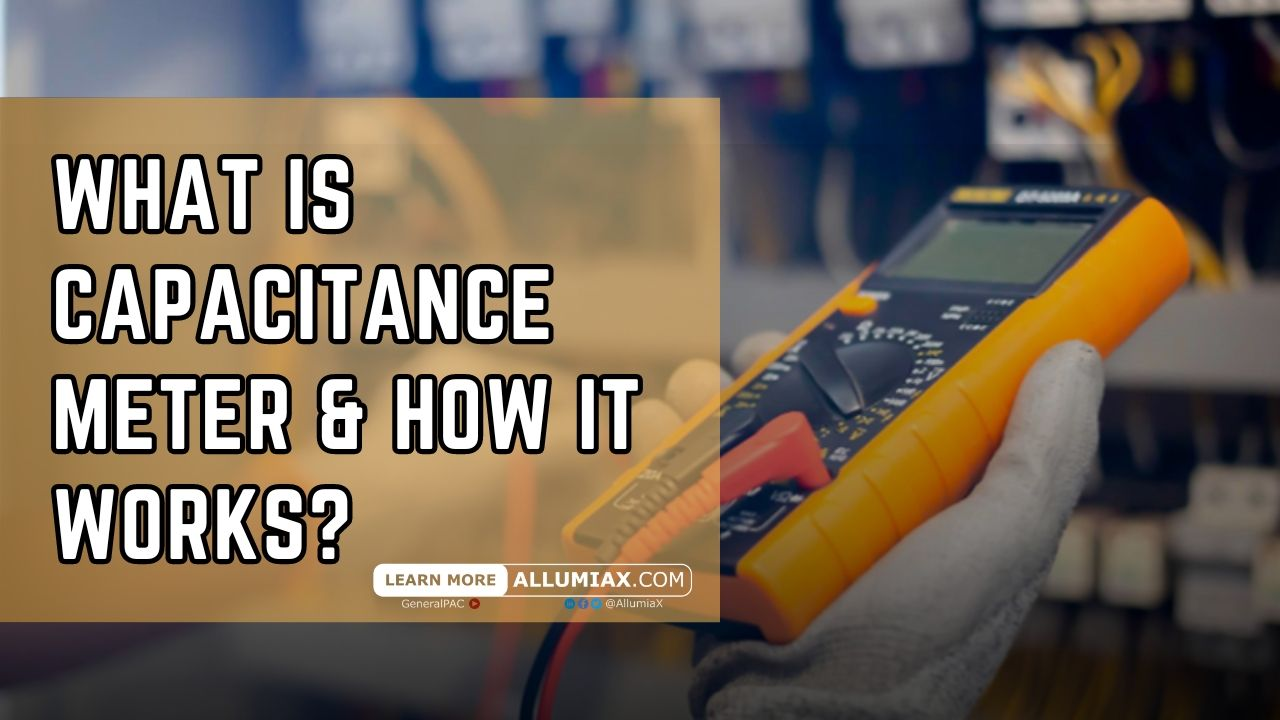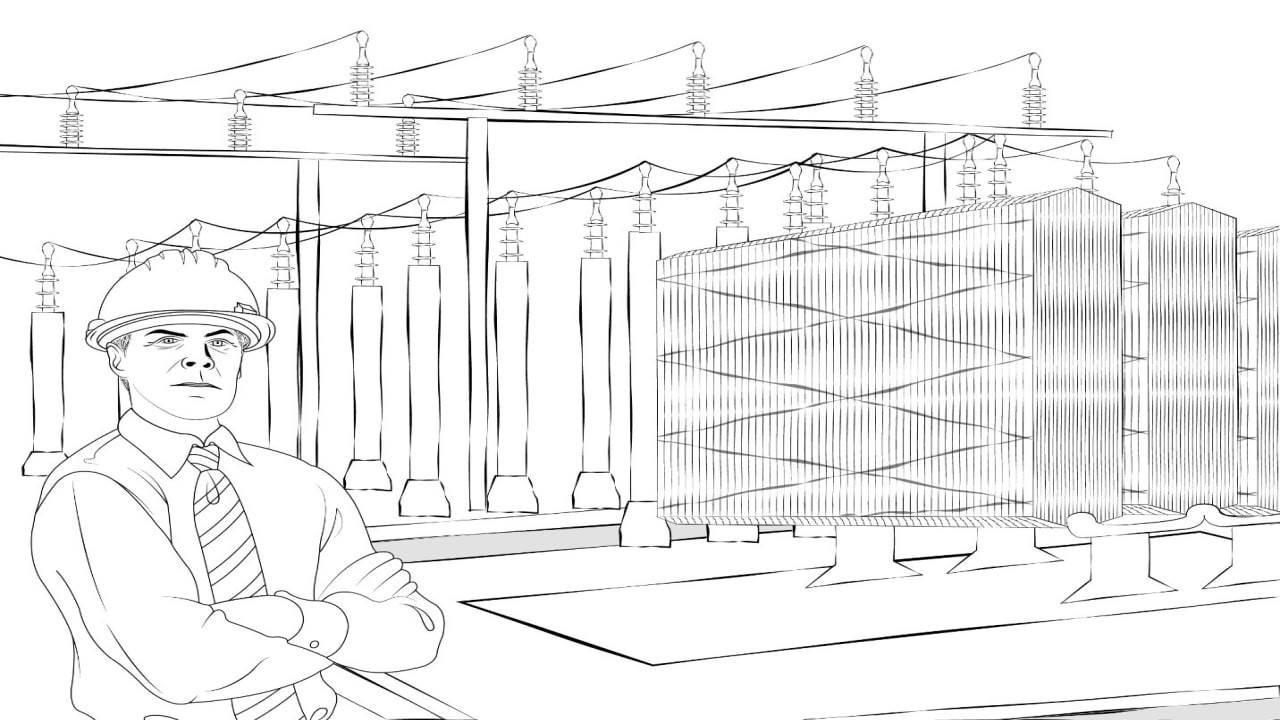(206) 687-4009 | [email protected]

# Capacitance Meter | What It Is, How It Works?Last updated: August 13th, 2023

## Introduction to Capacitance Meters

A capacitance meter is an electronic device used to measure capacitance. Capacitance is a property of an electronic component that determines the component's ability to store charge in a circuit. Capacitance meters can measure capacitance values from a few picofarads (pF) to a few farads (F).

Key features of capacitance meters include:

Accuracy: Capacitance meters are capable of providing highly accurate capacitance measurements, which is critical for many electronic and electrical applications.

Wide range: Capacitance meters can typically measure capacitance values from picofarads (pF) to millifarads (mF) and even farads (F), so they can be adapted to a wide variety of measurement needs.

Ease of Use: Many capacitance meters are designed to be user-friendly, with easy-to-read displays and even automatic range selection, making it easy for the user to make measurements.

Flexibility: Many capacitance meters can measure other electronic parameters such as resistance, inductance, voltage and current, making them more flexible in practical applications.

Portability: Many capacitance meters are designed to be portable, making it easy to take measurements in the field.

## Understanding capacitance

1. Explain capacitance and its role in electronics: Capacitance is a physical quantity used to measure the ability of a circuit element to store and release electrical energy. The unit of capacitance is the farad (F). In a capacitor, capacitance is created by the difference in charge between two conductors, often called plates. The space between these two conductors is filled with a dielectric (such as air, glass, or ceramic). Capacitance has roles in electronics such as filtering and smoothing, coupling and decoupling, tuning, and energy storage.
2. Unit of Measurement of Capacitance (Farad): The Farad is the International System of Units (SI) unit of capacitance. This unit is named after the British physicist Michael Faraday, who made significant contributions to the field of electromagnetism.

A capacitor of 1 farad (F) will store 1 coulomb (C) of charge when a voltage of 1 volt (V) is applied across its terminals. In other words, if a capacitor has a capacitance of 1 farad, it will store 1 coulomb of charge when its voltage is increased by 1 volt.

However, the farad is a very large unit, and for most practical applications it is common to use fractional units of the farad, such as the microfarad (uF, 10^-6F), nanofarad (nF, 10^-9F), or picofarad (pF, 10^-12F). These units are more appropriate for describing the capacitance of common electronic components such as capacitors.

## Functions of Capacitance Meter

Measuring capacitance: This is the basic function of a capacitance meter. It can measure capacitance values from picofarads (pF) to millifarads (mF) and even farads (F).

Measuring other electronic parameters: Many capacitance meters can also measure other electronic parameters such as resistance, inductance, voltage and current, which makes them more flexible in practical applications.

Fault detection: Capacitance meters can be used to detect whether a capacitor is faulty. For example, if the measured capacitance of a capacitor is too different from its nominal value, the capacitor may be damaged.

Data storage and readout: Many advanced capacitance meters have data storage and readout features that allow you to save your measurements for later viewing or analysis.

Automatic range selection: Some capacitance meters have an automatic range selection function, which can automatically select the most suitable measurement range, making the measurement more accurate and convenient.

## Types of Capacitance Meters

1. Analog Capacitance Meters: Analog capacitance meters use analog techniques (e.g., pointers and dials) to display measurements. These types of capacitance meters are usually cheaper, but their measurement accuracy and resolution are usually lower than those of digital capacitance meters. However, they can easily provide continuously varying readings, which may be necessary in some applications.
2. Digital Capacitance Meters: Digital capacitance meters use a digital display (e.g., LCD) to show measurement results. These types of capacitance meters typically have higher measurement accuracy and resolution than analog capacitance meters. In addition, they usually have more features such as automatic range selection, data storage and reading, and measurement of other electronic parameters (such as resistance, inductance, voltage, and current). However, digital capacitance meters are usually more expensive than analog capacitance meters.

## How Does a Capacitance Meter Work?

The working principle of a capacitance meter is based on a simple physical concept: when a capacitor is charged and discharged, the flow of charge generates a current that is proportional to the capacitance value of the capacitor.

The following are the basic steps in the operation of a capacitance meter:

1. The device applies a known voltage to the capacitor. This causes the capacitor to start charging.
2. The capacitance meter measures the time it takes for the capacitor to charge or discharge. This time is proportional to the capacitance value of the capacitor. 3.
3. The capacitance meter calculates the capacitance value of the capacitor by comparing this time to a known reference value (usually the charging or discharging time of an internal reference capacitor).
4. Finally, the capacitance meter displays this calculated capacitance value.

## Applications of Capacitance Meters

1. Electronic equipment manufacturing: when manufacturing electronic equipment, capacitance meters can be used to measure and verify the capacitance values of capacitors to ensure they meet design specifications.
2. Electronic equipment maintenance and troubleshooting: when problems arise with electronic equipment, capacitance meters can be used to check whether a capacitor has been damaged and help determine the source of the problem.
3. R&D and experimentation: In scientific research and product development, capacitance meters can be used to measure and compare the capacitive properties of different materials or designs.
4. Education and Training: Capacitance meters are commonly used to demonstrate and teach capacitance concepts and measurements in education and training in electronics and electrical engineering.
5. Industrial applications: In certain industrial applications, such as power systems, motor control and power electronics, capacitance meters can be used to measure and monitor the performance of capacitors to ensure proper system operation.
Close